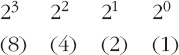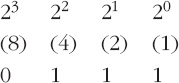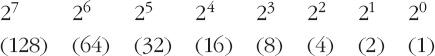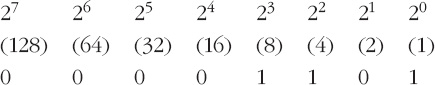Representing and manipulating data in computers

This free course is available to start right now. Review the full course description and key learning outcomes and create an account and enrol if you want a free statement of participation.

Free course

# 2.2.3 Positive integers: converting denary numbers to binary

If computers encode the denary numbers of the everyday world as binary numbers, then clearly there needs to be conversion from denary to binary and vice versa. You have just seen how to convert binary numbers to denary, because I did a couple of examples to show you how binary numbers ‘work’. But how can denary numbers be converted to binary? I'll show you by means of an example.

## Example 1

Convert 219 to an 8-bit binary number.

First write out the weightings of an 8-bit number:

 27 26 25 24 23 22 21 20 (128) (64) (32) (16) (8) (4) (2) (1)

Now decide whether the given number, 219, is larger than or equal to the largest weighting, 128. If the given number is larger than or equal to the weighting, record a 1 in the ‘128’ column and subtract the weighting from the number. But if the given number is smaller than the weighting, record a 0 in the ‘128’ column.

Here 219 is bigger than 128. Hence a 1 goes into the ‘128’ column (there is one ‘128’ in 219). This is shown below. The result of the subtraction 219 − 128 is 91.

 27 26 25 24 23 22 21 20 (128) (64) (32) (16) (8) (4) (2) (1) 1

Now decide whether the result of the subtraction, 91, is larger than or equal to than the next-lower weighting, 64. (I'll just call the result of the subtraction ‘the new number’.) If the new number is larger than or equal to the weighting, record a 1 in the ‘64’ column and subtract the weighting from the new number. If the new number is smaller than the weighting, record a 0 in the ‘64’ column.

Here 91 is larger than 64. Hence a 1 goes into the ‘64’ column (there is one ‘64’ in 91). This is shown below. The result of the subtraction 91 − 64 is 27.

 27 26 25 24 23 22 21 20 (128) (64) (32) (16) (8) (4) (2) (1) 1 1

Now decide whether the new number, 27, is larger than or equal to the next-lower weighting, 32. If the new number is larger than or equal to the weighting, record a 1 in the ‘32’ column and subtract the weighting from the new number. If the new number is smaller than the weighting, record a 0 in the ‘32’ column.

Here 27 is smaller than 32. Hence a 0 goes into the ‘32’ column (there are no ‘32s’ in 27):

 27 26 25 24 23 22 21 20 (128) (64) (32) (16) (8) (4) (2) (1) 1 1 0

Now decide whether the number brought forward, 27, is larger than or equal to the next-lower weighting, 16. If the number brought forward is larger than or equal to the weighting, record a 1 in the ‘16’ column and subtract the weighting from the new number. If the number brought forward is smaller than the weighting, record a 0 in the ‘16’ column.

Here 27 is larger than 16. Hence a 1 goes into the ‘16’ column (there is one ‘16’ in 27). This is shown below. The result of the subtraction 27 − 16 is 11.

 27 26 25 24 23 22 21 20 (128) (64) (32) (16) (8) (4) (2) (1) 1 1 0 1

The process continues for the last four remaining weightings, and you can check for yourself that the final result is:

 27 26 25 24 23 22 21 20 (128) (64) (32) (16) (8) (4) (2) (1) 1 1 0 1 1 0 1 1

The binary number can now be read off; it is 1101 1011.

Sometimes the first bit, or the first few bits, turn out to be 0 – as would be the case if, say, denary 6 was being converted to an 8-bit number. These ‘leading zeros’ must be included in the resulting binary number, otherwise it would not be 8 bits long. So, for example, 6 is 0000 0110 in 8-bit representation.

The same principles as those illustrated in the example above apply to other word lengths; the important point is to start the comparison-and-subtraction process with the weighting of the most-significant bit for the word length you are using.

## Activity 6 (Self assessment)

• (a) Convert denary 7 into a 4-bit number.

• (b) Convert the following denary numbers into 8-bit numbers:

• (i) 120

• (ii) 13

(a) Here the weightings are:8, so a 0 goes into the ‘8’ column.

Going on to the next-lower weighting, 4: 7 is larger than 4, so a 1 goes into the ‘4’ column. The subtraction is 7 − 4, which is 3.

Continuing like this gives:so the binary number is 0111.

(b) Here the weightings are those given in Example 1:(i) 120 is smaller than 128, so 0 goes into the ‘128’ column. 120 is larger than 64, so a 1 goes into the ‘64’ column. The subtraction 120 − 64 gives 56. 56 is larger than 32, so a 1 goes into the ‘32’ column. The subtraction 56 − 32 gives 24. And so on. The result is:so the binary number is 0111 1000.

(ii) Similarly, appropriate comparisons and subtractions here give:so the binary number is 0000 1101.

## Activity 7 (Exploratory)

Is there more than one possible result of converting a denary number into a binary number? Look back at the conversion of 219 (my example) and of 7, 120 and 13 (Activity 6) to help you to decide.

### Discussion

There is only one possible pattern of 1s and 0s in binary that equals a given denary number. You can alter things slightly by saying whether you want a 4-bit, 8-bit, 16-bit, etc., word (for example denary 7 is 0111 in a 4-bit word, but 0000 0111 in an 8-bit word), but the essence of the pattern stays the same.

This is because there is only one possible outcome of the comparison at each stage of the conversion process: either the number you are working with is larger than or equal to the next weighting or it is smaller than the next weighting. There is no possible ambiguity. Hence the pattern of 1s and 0s is fully defined and only one appropriate pattern can exist.

In conclusion, then, if the denary number to be encoded is a positive integer (a positive whole number), then the number is converted to binary form, as just described, so that it can be stored or manipulated in the computer. And after manipulation the result can be converted back to denary for reporting the outcome to the user. In the kitchen scales, for example, the weight is displayed as a denary number on the display.

## Box 1: Distinguishing between binary and denary numbers

Very often it's obvious whether a given number is binary or denary: 2975 is denary, and 0011 1010 is very likely (though not absolutely certain) to be binary. But is the number 1001 binary or denary?

One way of distinguishing clearly between binary and denary numbers is to write a subscript 2 at the end of a binary number and a subscript 10 at the end of a denary number. Thus

10012

is binary because of the subscript 2 at the end, whereas

100110

is denary because of the subscript 10 at the end.

Sometimes binary numbers are described as being ‘to base 2’. This fits with the subscript 2 at the end of the number. Similarly, denary numbers can be described as being ‘to base 10’, and this fits with the subscript 10 at the end.

You will meet the subscript convention on occasions in the rest of the course.

T224_2